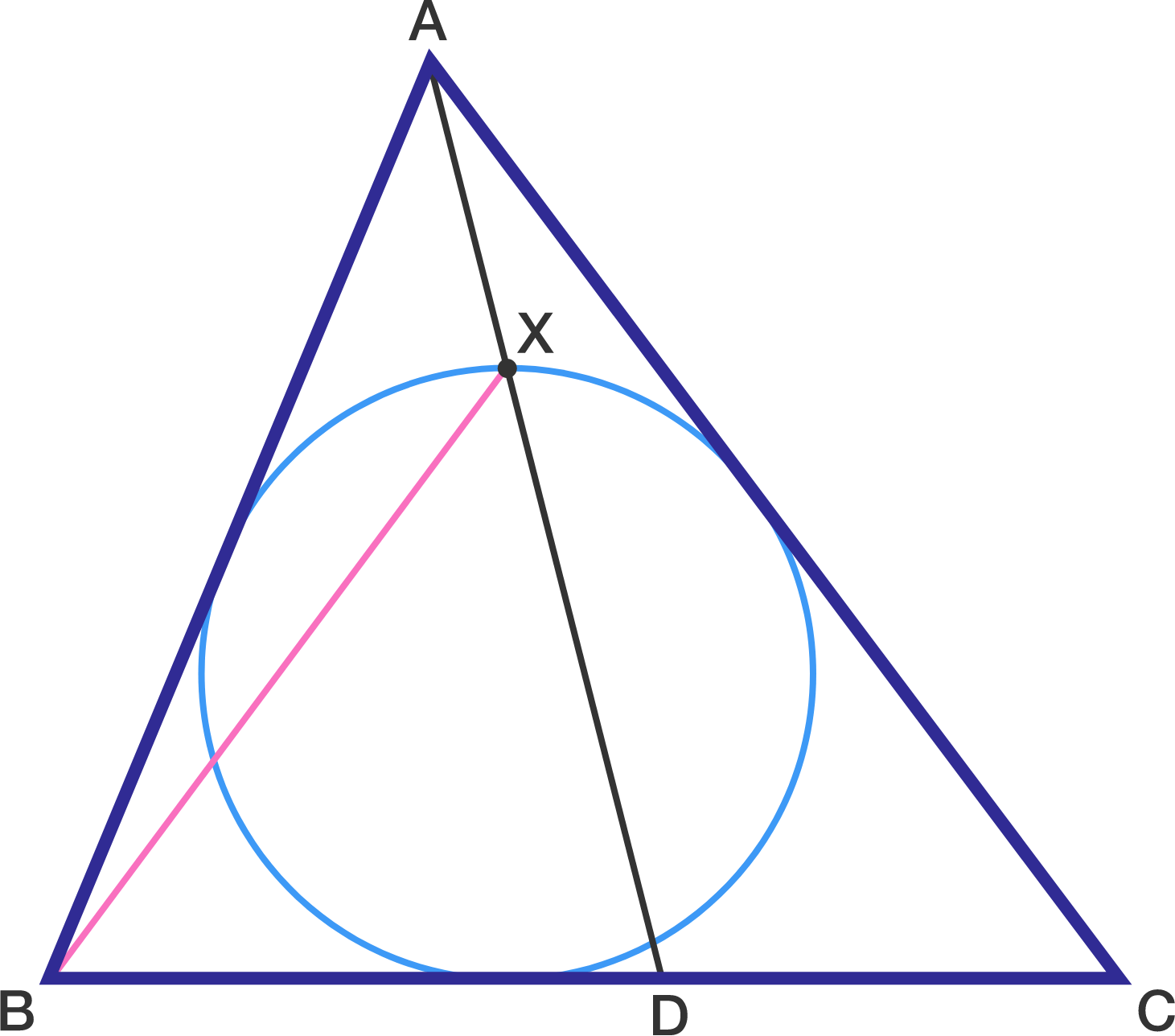# A Strange Cevian

Geometry Level 2Triangle $ABC$ is such that $AB = 13, BC = 14,$ and $CA = 15.$ A point $D$ on $BC$ is placed such that $AB + BD = AC + CD.$ Let $X$ be the intersection of $AD$ with the incircle of $ABC$ closest to $A.$ Find the length of $BX.$

×